Q-Chem 4.3 User’s Manual

# 9.1 Equilibrium Geometries and Transition Structures

Molecular potential energy surfaces rely on the Born-Oppenheimer separation of nuclear and electronic motion. Minima on such energy surfaces correspond to the classical picture of equilibrium geometries and first-order saddle points for transition structures. Both equilibrium and transition structures are stationary points and therefore the energy gradients will vanish. Characterization of the critical point requires consideration of the eigenvalues of the Hessian (second derivative matrix). Equilibrium geometries have Hessians whose eigenvalues are all positive. Transition structures, on the other hand, have Hessians with exactly one negative eigenvalue. That is, a transition structure is a maximum along a reaction path between two local minima, but a minimum in all directions perpendicular to the path.

The quality of a geometry optimization algorithm is of major importance; even the fastest integral code in the world will be useless if combined with an inefficient optimization algorithm that requires excessive numbers of steps to converge. Thus, Q-Chem incorporates the most advanced geometry optimization features currently available through Jon Baker’s Optimize package (see Appendix A), a product of over ten years of research and development.

The key to optimizing a molecular geometry successfully is to proceed from the starting geometry to the final geometry in as few steps as possible. Four factors influence the path and number of steps:

• starting geometry

• optimization algorithm

• quality of the Hessian (and gradient)

• coordinate system

Q-Chem controls the last three of these, but the starting geometry is solely determined by the user, and the closer it is to the converged geometry, the fewer optimization steps will be required. Decisions regarding the optimizing algorithm and the coordinate system are generally made by the Optimize package to maximize the rate of convergence. Users are able to override these decisions, but in general, this is not recommended.

Another consideration when trying to minimize the optimization time concerns the quality of the gradient and Hessian. A higher quality Hessian (i.e., analytical vs. approximate) will in many cases lead to faster convergence and hence, fewer optimization steps. However, the construction of an analytical Hessian requires significant computational effort and may outweigh the advantage of fewer optimization cycles. Currently available analytical gradients and Hessians are summarized in Table 9.1.

 Level of Theory Analytical Maximum Angular Analytical Maximum Angular (Algorithm) Gradients Momentum Type Hessian Momentum Type DFT ✓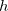✓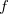HF ✓✓ROHF ✓✗ MP2 ✓✗ (V)OD ✓✗ (V)QCCD ✓✗ CIS (except RO) ✓✓CFMM ✓✗
Table 9.1: Gradients and Hessians currently available for geometry optimizations with maximum angular momentum types for analytical derivative calculations (for higher angular momentum, derivatives are computed numerically). Analytical Hessian is not yet available to tau-dependent functionals, such as BMK, M05 and M06 series.

Features of Q-Chem’s geometry and transition-state optimization capabilities include:

• Cartesian, Z-matrix or internal coordinate systems

• Eigenvector Following (EF) or GDIIS algorithms

• Constrained optimizations

• Equilibrium structure searches

• Transition structure searches

• Initial Hessian and Hessian update options

• Reaction pathways using intrinsic reaction coordinates (IRC)

• Optimization of minimum-energy crossing points (MECPs) along conical seams

## 9.1.1 Job Control

Note:  Users input starting geometry through the $molecule keyword. Users must first define what level of theory is required. Refer back to previous sections regarding enhancements and customization of these features. METHOD (or EXCHANGE and CORRELATION, if required) and BASIS$rem variables must be set.

The remaining $rem variables are those specifically relating to the Optimize package. JOBTYPE  Specifies the calculation. TYPE:  STRING DEFAULT:  Default is single-point, which should be changed to one of the following options. OPTIONS:  OPT Equilibrium structure optimization. TS Transition structure optimization. RPATH Intrinsic reaction path following. RECOMMENDATION:  Application-dependent. GEOM_OPT_HESSIAN  Determines the initial Hessian status. TYPE:  STRING DEFAULT:  DIAGONAL OPTIONS:  DIAGONAL Set up diagonal Hessian. READ Have exact or initial Hessian. Use as is if Cartesian, or transform if internals. RECOMMENDATION:  An accurate initial Hessian will improve the performance of the optimizer, but is expensive to compute. GEOM_OPT_COORDS  Controls the type of optimization coordinates. TYPE:  INTEGER DEFAULT:  -1 OPTIONS:  0 Optimize in Cartesian coordinates. 1 Generate and optimize in internal coordinates, if this fails abort. -1 Generate and optimize in internal coordinates, if this fails at any stage of the optimization, switch to Cartesian and continue. 2 Optimize in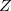-matrix coordinates, if this fails abort. -2 Optimize in-matrix coordinates, if this fails during any stage of the optimization switch to Cartesians and continue. RECOMMENDATION:  Use the default; delocalized internals are more efficient. GEOM_OPT_TOL_GRADIENT  Convergence on maximum gradient component. TYPE:  INTEGER DEFAULT:  300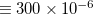tolerance on maximum gradient component. OPTIONS:Integer value (tolerance =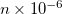). RECOMMENDATION:  Use the default. To converge GEOM_OPT_TOL_GRADIENT and one of GEOM_OPT_TOL_DISPLACEMENT and GEOM_OPT_TOL_ENERGY must be satisfied. GEOM_OPT_TOL_DISPLACEMENT  Convergence on maximum atomic displacement. TYPE:  INTEGER DEFAULT:  1200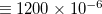tolerance on maximum atomic displacement. OPTIONS:Integer value (tolerance =). RECOMMENDATION:  Use the default. To converge GEOM_OPT_TOL_GRADIENT and one of GEOM_OPT_TOL_DISPLACEMENT and GEOM_OPT_TOL_ENERGY must be satisfied. GEOM_OPT_TOL_ENERGY  Convergence on energy change of successive optimization cycles. TYPE:  INTEGER DEFAULT:  100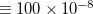tolerance on maximum gradient component. OPTIONS:Integer value (tolerance = value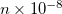). RECOMMENDATION:  Use the default. To converge GEOM_OPT_TOL_GRADIENT and one of GEOM_OPT_TOL_DISPLACEMENT and GEOM_OPT_TOL_ENERGY must be satisfied. GEOM_OPT_MAX_CYCLES  Maximum number of optimization cycles. TYPE:  INTEGER DEFAULT:  50 OPTIONS:User defined positive integer. RECOMMENDATION:  The default should be sufficient for most cases. Increase if the initial guess geometry is poor, or for systems with shallow potential wells. GEOM_OPT_PRINT  Controls the amount of Optimize print output. TYPE:  INTEGER DEFAULT:  3 Error messages, summary, warning, standard information and gradient print out. OPTIONS:  0 Error messages only. 1 Level 0 plus summary and warning print out. 2 Level 1 plus standard information. 3 Level 2 plus gradient print out. 4 Level 3 plus Hessian print out. 5 Level 4 plus iterative print out. 6 Level 5 plus internal generation print out. 7 Debug print out. RECOMMENDATION:  Use the default. ## 9.1.2 Customization GEOM_OPT_SYMFLAG  Controls the use of symmetry in Optimize. TYPE:  INTEGER DEFAULT:  1 OPTIONS:  1 Make use of point group symmetry. 0 Do not make use of point group symmetry. RECOMMENDATION:  Use default. GEOM_OPT_MODE  Determines Hessian mode followed during a transition state search. TYPE:  INTEGER DEFAULT:  0 OPTIONS:  0 Mode following off.Maximize along mode. RECOMMENDATION:  Use default, for geometry optimizations. GEOM_OPT_MAX_DIIS  Controls maximum size of subspace for GDIIS. TYPE:  INTEGER DEFAULT:  0 OPTIONS:  0 Do not use GDIIS. -1 Default size = min(NDEG, NATOMS, 4) NDEG = number of molecular degrees of freedom.Size specified by user. RECOMMENDATION:  Use default or do not settoo large. GEOM_OPT_DMAX  Maximum allowed step size. Value supplied is multiplied by 10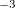. TYPE:  INTEGER DEFAULT:  300 = 0.3 OPTIONS:User-defined cutoff. RECOMMENDATION:  Use default. GEOM_OPT_UPDATE  Controls the Hessian update algorithm. TYPE:  INTEGER DEFAULT:  -1 OPTIONS:  -1 Use the default update algorithm. 0 Do not update the Hessian (not recommended). 1 Murtagh-Sargent update. 2 Powell update. 3 Powell/Murtagh-Sargent update (TS default). 4 BFGS update (OPT default). 5 BFGS with safeguards to ensure retention of positive definiteness (GDISS default). RECOMMENDATION:  Use default. GEOM_OPT_LINEAR_ANGLE  Threshold for near linear bond angles (degrees). TYPE:  INTEGER DEFAULT:  165 degrees. OPTIONS:User-defined level. RECOMMENDATION:  Use default. FDIFF_STEPSIZE  Displacement used for calculating derivatives by finite difference. TYPE:  INTEGER DEFAULT:  100 Corresponding to 0.001 . For calculating second derivatives. OPTIONS:Use a step size of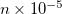. RECOMMENDATION:  Use default, unless on a very flat potential, in which case a larger value should be used. See FDIFF_STEPSIZE_QFF for third and fourth derivatives. ## 9.1.3 Example Example 9.188 As outlined, the rate of convergence of the iterative optimization process is dependent on a number of factors, one of which is the use of an initial analytic Hessian. This is easily achieved by instructing Q-Chem to calculate an analytic Hessian and proceed then to determine the required critical point $molecule
0  1
O
H  1  oh
H  1  oh 2 hoh

oh  = 1.1
hoh = 104
$end$rem
JOBTYPE    freq   Calculate an analytic Hessian
METHOD     hf
BASIS      6-31g(d)
$end$comment
Now proceed with the Optimization making sure to read in the analytic
Hessian (use other available information too).
$end @@@$molecule
$end$rem